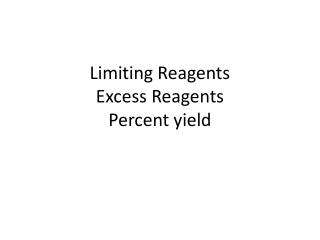DownloadDownload PresentationLimiting Reagents Excess Reagents Percent yield

# Limiting Reagents Excess Reagents Percent yield

Download Presentation## Limiting Reagents Excess Reagents Percent yield

- - - - - - - - - - - - - - - - - - - - - - - - - - - E N D - - - - - - - - - - - - - - - - - - - - - - - - - - -
##### Presentation Transcript

1. Limiting ReagentsExcess ReagentsPercent yield

2. Limiting Reagents • The limiting reagent is the reactant that runs out first • Limits the amount of product that can be formed • Take both reactants to the same product • The answer is always the smallest amount of product

3. Example • Potassium superoxide, KO2 is used in rebreathing gas masks to generate oxygen. 4 KO2(s) + 2H2O(l)g 4KOH + 3O2(g) If a reaction vessel contains 0.25 mol KO2 and 0.15 mol H2O what is the limiting reagent? How many moles of oxygen can be produced? • Take both starting amounts to moles of oxygen, the limiting reagent is the one that produced the smallest amount. 0.25 mol KO2 | 3 mol O2= 0.19 mol O2 | 4 mol KO2 0.15 mol H2O | 3 mol O2= 0.23 mol O2 | 2 mol H2O This is the smallest amount of O2 therefore KO2 is the limiting reagent and this is the amount of O2 produced.

4. Excess Reagent • The reagent (reactant) that is left over once the reaction stops. • Extra reactant • To determine the amount of left over (excess) reagent • Take both reactants to same product. • Find the difference between the two amounts of products • Use that a the starting number and convert back to the excess reagent.

5. Example • Potassium superoxide, KO2 is used in rebreathing gas masks to generate oxygen. 4 KO2(s) + 2H2O(l)g 4KOH + 3O2(g) If a reaction vessel contains 0.25 mol KO2 and 0.15 mol H2O what is the excess reagent? How many moles were in excess? • Take both starting amounts to moles of oxygen, find the difference between the two amounts, convert back to the excess reagent 0.25 mol KO2 | 3 mol O2= 0.19 mol O2 | 4 mol KO2 0.15 mol H2O | 3 mol O2= 0.23 mol O2 | 2 mol H2O 0.23 – .19 molO2 | 2 mol H2O = 0.03 mol H2O | 3 mol O2 This is the smallest amount of O2 therefore KO2 is the limiting reagent and H2O is the excess reagent. This is the amount of excess

6. Percent Yield • Is found using the formula: Actual Theoretical • The actual yield is what is actually produced in the lab • The theoretical yield is what is supposed to be formed based on stoichiometry *100

7. Example • Potassium superoxide, KO2 is used in rebreathing gas masks to generate oxygen. 4 KO2(s) + 2H2O(l)g 4KOH + 3O2(g) If a reaction vessel contains 0.25 mol KO2 and 0.15 mol H2O what is the limiting reagent? How many moles of oxygen can be produced? In the lab 0.15 mol of O2 were actually produced, what is the percent yield? • Take both starting amounts to moles of oxygen, the limiting reagent is the one that produced the smallest amount. Divide actual yield by the theoretical yield based on limiting reagent. 0.25 mol KO2 | 3 mol O2= 0.19 mol O2 | 4 mol KO2 0.15 mol H2O | 3 mol O2= 0.23 mol O2 | 2 mol H2O For % yield, 0.15 / 0.19 *100 = 79% This is not the greatest % yield, this of the percentage as your test score. This is the smallest amount of O2 therefore KO2 is the limiting reagent and this is the amount of O2 produced.